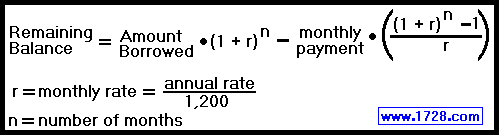Calculating the Interest, Equity and Balance of a Loan

 To see how monthly payments affect the equity, interest and balance of a loan, let's use an example. We want to take a loan for \$2,500.00 for 3 years at 7 per cent interest. First, using the calculator located here, we find the monthly payment is \$77.19. The next thing to do is calculate the 7 per cent interest rate as a monthly rate.           .07 ÷ 12 = 0.005833333333...
 Let's calculate the results after the first payment.     Interest Paid = Previous Balance * Monthly Interest Rate Interest Paid = \$2,500.00 * 0.005833333333 Interest Paid = 14.5833333333 or \$14.58 (rounded)     Equity = Monthly Payment -Interest Paid Equity = \$77.19 -\$14.58 = \$62.61     New Balance = Previous Balance -Equity New Balance = \$2,500.00 -\$62.61 = \$2,437.39 ******************************************************************** ********************************************************************
 Now for the second payment. Interest Paid = \$2,437.39 * 0.005833333333 Interest Paid = \$14.218108333 or \$14.22 (rounded) Equity = \$77.19 -\$14.22 = \$62.97 New Balance = \$2,437.39 -\$62.97 = \$2,374.42 ******************************************************************** ********************************************************************

Now for the third payment.

Interest Paid = \$2,374.42 * 0.005833333333
Interest Paid = 13.85078333254 or \$13.85 (rounded)

Equity = \$77.19 -\$13.85 = \$63.34

New Balance = \$2,374.42 -\$62.97 = \$2,311.08

The above calculations are summarized in this table.

 Payment # Payment Amount Interest Paid Equity *** Balance *** *** ****** ****** ****** \$2,500.00 1 \$77.19 \$14.58 \$62.61 \$2,437.39 2 \$77.19 \$14.22 \$62.97 \$2,374.42 3 \$77.19 \$13.85 \$63.34 \$2,311.08
If we were to make line 2, the sum of lines 1 and 2 and
if line 3 was the sum of lines 1, 2 and 3 the results would be:
 Payment # Payment Total Interest Total You Own *** Balance *** *** ****** ****** ****** \$2,500.00 1 \$77.19 \$14.58 \$62.61 \$2,437.39 2 \$154.38 \$28.80 \$125.58 \$2,374.42 3 \$231.57 \$42.65 \$188.92 \$2,311.08

That's fine if you just want to calculate a loan's balance month by month but is there a formula for calculating a loan balance after several months have passed?
Yes, and here's the formula:Now let's try that third month payment using the above formula.

Remaining Balance = \$2,500.00 * (1.005833333333)3 - 77.19 * [((1.005833333333)3-1) ÷ .005833333333]

Remaining Balance = \$2,500.00 * 1.0176022818 -77.19 * (0.0176022818 ÷ .005833333333)

Remaining Balance = 2,544.0057045692 - 77.19 * 3.017534023

Remaining Balance = 2,544.0057045692 - 232.9234512377

Remaining Balance = 2,311.08

It's much easier with that formula isn't it?

This is the way the calculator located here performs its calculations.

R E T U R N     T O     H O M E     P A G E